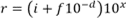# 3.3.2 Number Tokens

``` INTEGER = integer-literal ["%" / "&" / "^"]
integer-literal = decimal-literal / octal-literal / hex-literal
decimal-literal = 1*decimal-digit
octal-literal = "&" [%x004F / %x006F] 1*octal-digit    ; & or &o or &O
hex-literal = "&" (%x0048 / %x0068) 1*hex-digit   ; &h or &H
octal-digit = "0" / "1" / "2" / "3" / "4" / "5" / "6" / "7"
decimal-digit = octal-digit / "8" / "9"
hex-digit = decimal-digit / %x0041-0046 / %x0061-0066 ;A-F / a-f
```

Static Semantics

• The <decimal-digit>, <octal-digit>, and <hex-digit> sequences are interpreted as unsigned integer values represented respectively in decimal, octal, and hexadecimal notation.

• Each <INTEGER> has an associated constant data value (section 2.1). The data value, value type (section 2.1) and declared type (section 2.2) of the constant is defined by the following table (if the Valid column shows No, this <INTEGER> is invalid):

Positive <INTEGER> value in the range

Type Suffix

Valid <INTEGER>?

Declared Type

Value Type

Signed Data Value

Decimal

0 ≤ n ≤ 32767

None

Yes

Integer

Integer

n

Decimal

0 ≤ n ≤ 32767

"%"

Yes

Integer

Integer

n

Decimal

0 ≤ n ≤ 32767

"&"

Yes

Long

Integer

n

Decimal

0 ≤ n ≤ 32767

"^"

Yes

LongLong

Integer

n

Octal

0 ≤ n ≤ &o77777

None

Yes

Integer

Integer

n

Octal

0 ≤ n ≤ &o77777

"%"

Yes

Integer

Integer

n

Octal

0 ≤ n ≤ &o77777

"&"

Yes

Long

Integer

n

Octal

0 ≤ n ≤ &o77777

"^"

Yes

LongLong

Integer

n

Octal

&o100000 ≤ n ≤ &o177777

None

Yes

Integer

Integer

n – 65,536

Octal

&o100000 ≤ n ≤ &o177777

"%"

Yes

Integer

Integer

n – 65,536

Octal

&o100000 ≤ n ≤ &o177777

"&"

Yes

Long

Integer

n

Octal

&o100000 ≤ n ≤ &o177777

"^"

Yes

LongLong

Integer

n

Hex

0 ≤ n ≤ &H7FFF

None

Yes

Integer

Integer

n

Hex

0 ≤ n ≤ &H7FFF

"%"

Yes

Integer

Integer

n

Hex

0 ≤ n ≤ &H7FFF

"&"

Yes

Long

Integer

n

Hex

0 ≤ n ≤ &H7FFF

"^"

Yes

LongLong

Integer

n

Hex

&H8000 ≤ n ≤ &HFFFF

None

Yes

Integer

Integer

n – 65,536

Hex

&H8000 ≤ n ≤ &HFFFF

"%"

Yes

Integer

Integer

n – 65,536

Hex

&H8000 ≤ n ≤ &HFFFF

"&"

Yes

Long

Integer

n

Hex

&H8000 ≤ n ≤ &HFFFF

"^"

Yes

LongLong

Integer

n

Decimal

32768 ≤ n ≤ 2147483647

None

Yes

Long

Long

n

Decimal

n ≥ 32768

"%"

No

Decimal

32768 ≤ n ≤ 2147483647

"&"

Yes

Long

Long

n

Decimal

32768 ≤ n ≤ 2147483647

"^"

Yes

LongLong

Long

n

Decimal

n ≥ 2147483647

None

(see note 1)

Double

Double

n# (see note 1)

Decimal

n ≥ 2147483647

"&"

No

Octal

&o200000 ≤ n ≤ &o17777777777

None

Yes

Long

Long

n

Octal

&o200000 ≤ n ≤ &o17777777777

"%"

No

Octal

&o200000 ≤ n ≤ &o17777777777

"&"

Yes

Long

Long

n

Octal

&o200000 ≤ n ≤ &o17777777777

"^"

Yes

LongLong

Long

n

Octal

&o20000000000 ≤ n ≤ &o37777777777

None

Yes

Long

Long

n – 4,294,967,296

Octal

&o20000000000 ≤ n ≤ &o37777777777

"%"

No

Octal

&o20000000000 ≤ n ≤ &o37777777777

"&"

Yes

Long

Long

n – 4,294,967,296

Octal

&o20000000000 ≤ n ≤ &o37777777777

"^"

Yes

LongLong

Long

n

Octal

n ≥ &o40000000000

None

No

Octal

n ≥ &o40000000000

"%"

No

Octal

n ≥ &o40000000000

"&"

No

Hex

&H8000 ≤ n ≤ &H7FFFFFFF

None

Yes

Long

Long

n

Hex

&H8000 ≤ n ≤ &H7FFFFFFF

"%"

No

Hex

&H8000 ≤ n ≤ &H7FFFFFFF

"&"

Yes

Long

Long

n

Hex

&H8000 ≤ n ≤ &H7FFFFFFF

"^"

Yes

LongLong

Long

n

Hex

&H80000000 ≤ n ≤ &H7FFFFFFFF

None

Yes

Long

Long

n – 4,294,967,296

Hex

&H80000000 ≤ n ≤ &H7FFFFFFFF

"%"

No

Hex

&H80000000 ≤ n ≤ &H7FFFFFFFF

"&"

Yes

Long

Long

n – 4,294,967,296

Hex

&H80000000 ≤ n ≤ &H7FFFFFFFF

"^"

Yes

LongLong

Long

n

Hex

n ≥ &H100000000

None

No

Hex

n ≥ &H100000000

"%"

No

Hex

n ≥ &H100000000

"&"

No

Decimal

2147483648 ≤ n ≤ 9223372036854775807

"^"

Yes

LongLong

LongLong

n

Decimal

n ≥ 9223372036854775808

"^"

Octal

&o40000000000 ≤ n ≤ &o1777777777777777777777

"^"

Yes

LongLong

LongLong

n - 232

Octal

n ≥ &o2000000000000000000000

Any

No

Hex

&H100000000 ≤ n ≤ &HFFFFFFFFFFFFFFFF

"^"

Yes

LongLong

LongLong

n - 232

Hex

n ≥ &H10000000000000000

Any

No

• It is statically invalid for a literal to have the declared type LongLong in an implementation that does not support 64-bit arithmetic.

```FLOAT = (floating-point-literal [floating-point-type-suffix] ) / (decimal-literal floating-point-type-suffix)
floating-point-literal = (integer-digits exponent) / (integer-digits "." [fractional-digits] [exponent]) / ( "." fractional-digits [exponent])

integer-digits = decimal-literal
fractional-digits = decimal-literal
exponent = exponent-letter  [sign] decimal-literal
exponent-letter = %x0044 / %x0045 / %x0064 / %x0065   ; D / E / d / e
sign = "+" / "-"
floating-point-type-suffix = "!" / "#" / "@"
```

Static Semantics

• <FLOAT> tokens represent either binary floating point or currency data values. The <floatingpoint-type-suffix> designates the declared type and value type of the data value associated with the token according to the following table:

<floating-point-type-suffix>

Declared Type and Value Type

Not present

Double

!

Single

#

Double

@

Currency

• Let i equal the integer value of <integer-digits>, f be the integer value of <fractional-digits>, d be the number of digits in <fractional-digits>, and x be the signed integer value of <exponent>. A <floating-point-literal> then represents a mathematical real number, r, according to this formula:• A <floating-point-literal> is invalid if its mathematical value is greater than the greatest mathematical value that can be represented using its declared type.

• If the declared type of <floating-point-literal> is Currency, the fractional part of r is rounded using Banker’s rounding (section 5.5.1.2.1.1) to 4 significant digits.